Next: Chromatic aberration Up: Paraxial Optics Previous: Thin Lenses

## Image Formation by Thin Lenses

There are two alternative methods of locating the image formed by a thin lens. Just as for spherical mirrors, the first method is graphical, and the second analytical.

The graphical method of locating the image formed by a thin lens involves drawing light-rays emanating from key points on the object, and finding where these rays are brought to a focus by the lens. This task can be accomplished using a small number of simple rules.

Consider a converging lens. It is helpful to define two focal points for such a lens. The first, the so-called image focus, denoted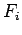, is defined as the point behind the lens to which all incident light-rays parallel to the optic axis converge after passing through the lens. This is the same as the focal point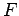defined previously. The second, the so-called object focus, denoted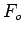, is defined as the position in front of the lens for which rays emitted from a point source of light placed at that position would be refracted parallel to the optic axis after passing through the lens. It is easily demonstrated that the object focusis as far in front of the optic centre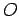of the lens as the image focusis behind. The distance from the optic centre to either focus is, of course, equal to the focal length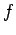of the lens. The image produced by a converging lens can be located using just three simple rules:

1. An incident ray which is parallel to the optic axis is refracted through the image focusof the lens.
2. An incident ray which passes through the object focusof the lens is refracted parallel to the optic axis.
3. An incident ray which passes through the optic centreof the lens is not refracted at all.
The last rule is only an approximation. It turns out that although a light-ray which passes through the optic centre of the lens does not change direction, it is displaced slightly to one side. However, this displacement is negligible for a thin lens.

Figure 80 illustrates how the image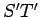of an object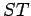placed in front of a converging lens is located using the above rules. In fact, the three rays, 1-3, emanating from the tip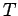of the object, are constructed using rules 1-3, respectively. Note that the image is real (since light-rays actually cross), inverted, and diminished.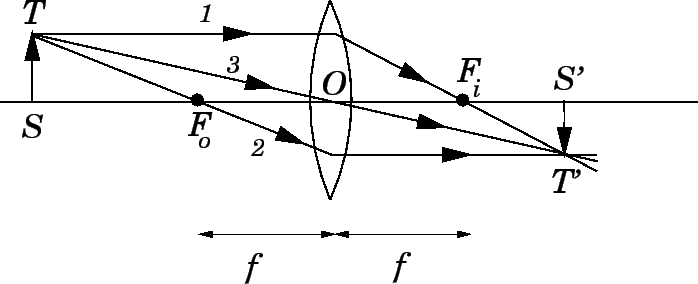Consider a diverging lens. It is again helpful to define two focal points for such a lens. The image focusis defined as the point in front of the lens from which all incident light-rays parallel to the optic axis appear to diverge after passing through the lens. This is the same as the focal pointdefined earlier. The object focusis defined as the point behind the lens to which all incident light-rays which are refracted parallel to the optic axis after passing through the lens appear to converge. Both foci are located a distancefrom the optic centre, whereis the focal length of the lens. The image produced by a diverging lens can be located using the following three rules:

1. An incident ray which is parallel to the optic axis is refracted as if it came from the image focusof the lens.
2. An incident ray which is directed towards the object focusof the lens is refracted parallel to the optic axis.
3. An incident ray which passes through the optic centreof the lens is not refracted at all.

Figure 81 illustrates how the imageof an objectplaced in front of a diverging lens is located using the above rules. In fact, the three rays, 1-3, emanating from the tipof the object, are constructed using rules 1-3, respectively. Note that the image is virtual (since light-rays do not actually cross), upright, and diminished.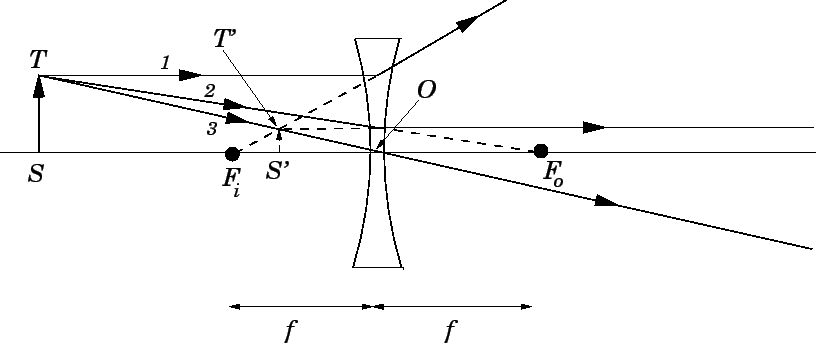Let us now investigate the analytical method. Consider an object of height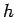placed a distance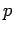in front of a converging lens. Suppose that a real image of height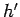is formed a distancebehind the lens. As is illustrated in Fig. 82, the image can be located using rules 1 and 3, discussed above.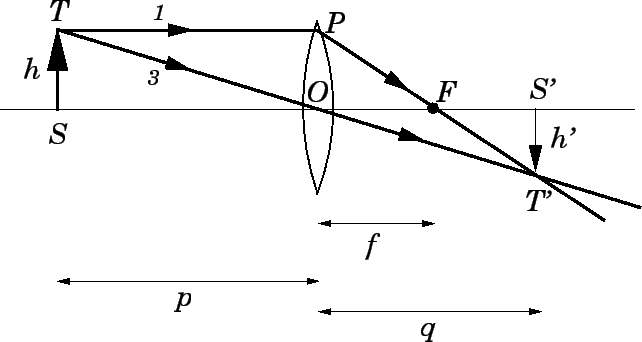Now, the right-angled triangles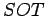and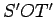are similar, so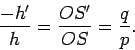(363)

Here, we have adopted the convention that the image heightis negative if the image is inverted. The magnification of a thin converging lens is given by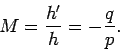(364)

This is the same as the expression (352) for the magnification of a spherical mirror. Note that we are again adopting the convention that the magnification is negative if the image is inverted.

The right-angled triangles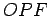and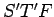are also similar, and so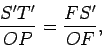(365)

or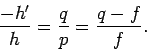(366)

The above expression can be rearranged to give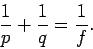(367)

Note that this is exactly the same as the formula (358) relating the image and object distances in a spherical mirror.

Although formulae (364) and (367) were derived for the case of a real image formed by a converging lens, they also apply to virtual images, and to images formed by diverging lenses, provided that the following sign conventions are adopted. First of all, as we have already mentioned, the focal lengthof a converging lens is positive, and the focal length of a diverging lens is negative. Secondly, the image distanceis positive if the image is real, and, therefore, located behind the lens, and negative if the image is virtual, and, therefore, located in front of the lens. It immediately follows, from Eq. (364), that real images are always inverted, and virtual images are always upright.

Table 7 shows how the location and character of the image formed by a converging lens depend on the location of the object. Here, the point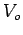is located on the optic axis two focal lengths in front of the optic centre, and the point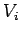is located on the optic axis two focal lengths behind the optic centre. Note the almost exact analogy between the image forming properties of a converging lens and those of a concave spherical mirror.

Table 7: Rules for image formation by converging lenses.
 Position of object Position of image Character of image At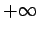AtReal, zero size BetweenandBetweenandReal, inverted, diminished AtAtReal, inverted, same size BetweenandBetweenand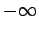Real, inverted, magnified AtAtBetweenandFromtoVirtual, upright, magnified AtAtVirtual, upright, same size

Table 8 shows how the location and character of the image formed by a diverging lens depend on the location of the object. Note the almost exact analogy between the image forming properties of a diverging lens and those of a convex spherical mirror.

Table 8: Rules for image formation by diverging lenses.
 Position of object Position of image Character of image At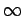AtVirtual, zero size BetweenandBetweenandVirtual, upright, diminished AtAtVirtual, upright, same size

Finally, let us reiterate the sign conventions used to determine the positions and characters of the images formed by thin lenses:

1. The heightof the image is positive if the image is upright, with respect to the object, and negative if the image is inverted.
2. The magnification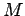of the image is positive if the image is upright, with respect to the object, and negative if the image is inverted.
3. The image distanceis positive if the image is real, and, therefore, located behind the lens, and negative if the image is virtual, and, therefore, located in front of the lens.
4. The focal lengthof the lens is positive if the lens is converging, so that the image focusis located behind the lens, and negative if the lens is diverging, so that the image focusis located in front of the lens.
Note that the front side of the lens is defined to be the side from which the light is incident.Next: Chromatic aberration Up: Paraxial Optics Previous: Thin Lenses
Richard Fitzpatrick 2007-07-14